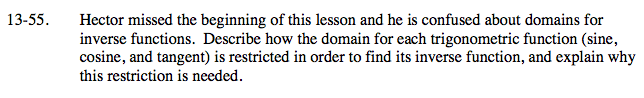### Home > A2C > Chapter 13 > Lesson 13.1.3 > Problem13-55

13-55.

Hector missed the beginning of this lesson and he is confused about domains for inverse functions. Describe how the domain for each trigonometric function (sine, cosine, and tangent) is restricted in order to find its inverse function, and explain why this restriction is needed. Homework Help ✎Think about the definition of a function. Is the inverse of a trigonometric function also a function?